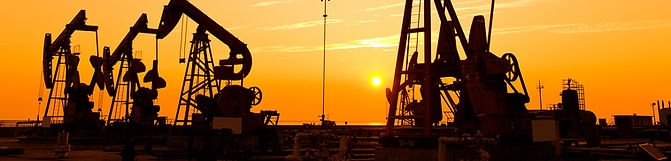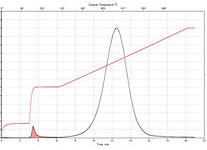top of page# Programmed Pyrolysis Definitions

## Definition List###### S1 (oil yield)

The amount of thermally freed hydrocarbons in the sample in milligrams hydrocarbons per gram of rock (mg HC/g rock).###### S2 (kerogen yield)

The amount of hydrocarbons generated through thermal cracking of non-volatile organic matter. S2 is an indication of the quantity of hydrocarbons that the rock has the potential of producing under increased burial and maturation. This is the kerogen yield. S2 measurements are reported in milligrams hydrocarbons per gram of rock (mg HC/g rock).###### S3 (organic carbon dioxide yield)

The amount of CO2 in milligrams CO2 per gram of rock produced during pyrolysis of kerogen (mg CO2/g rock). This is a measure of the organic carbon dioxide yield of the rock. S3 is an indication of the amount of oxygen in the kerogen.###### S3CO (organic carbon monoxide yield)

The amount of CO in milligrams CO per gram of rock produced during pyrolysis of kerogen (mg CO/g rock). This is a measure of the organic carbon monoxide pyrolysis yield of the rock.###### S4 (yield of organic carbon dioxide and carbon monoxide)

The amount of residual carbon, measured through the yield of carbon dioxide and carbon monoxide that is produced by oxidation after the completion of pyrolysis. It is measured in mg CO2/g rock and mg CO/g rock.###### S5 (yield of inorganic carbon dioxide)

Milligrams of carbon per gram or rock produced from the organic residue remaining after pyrolysis of kerogen is completed (mg C/g rock).###### Tmax (maturity)

The temperature at which the maximum generation of hydrocarbons from cracking of kerogen occurs during pyrolysis. This is the temperature at the top of the S2 peak. Measurements of Tmax are reported in °C. Tmax is an indicator of thermal maturity.###### TOC (total organic carbon)

measure of the Total Organic Carbon content of the rock in weight percent (wt. %).###### CC (Carbonate Carbon)

Measure of the inorganic carbon content of the rock and is derived from the mineral carbonate content of the rock (wt. %).###### Absolute Tmax for kinetics calculations

Measure of the inorganic carbon content of the rock and is derived from the mineral carbonate content of the rock (wt. %).

###### Plus the Following Interpretative Calculations:​measure of the rock’s capability to adsorb hydrocarbons; the higher the organic richness of a rock, the higher its AI. High organic content shales have a higher adsorptive capacity than low organic content carbonates. Adsorption Index is computed using the formula; AI  (wt. %)= 0.82 x TOC (wt. %).###### Oil Saturation Index (OSI)

measure of the normalized oil content and is computed using the formula; S1 (mg HC/g rock)/TOC (wt. %) x 100.###### Hydrogen Index (HI)

measure of the relative hydrogen abundance and is computed using the formula; HI = S2 (mg HC/g rock)/TOC (wt. %) x 100.###### Oxygen Index (OI)

measure of the relative oxygen abundance and is computed using the formula; OI = S3 (mg CO2/g rock)/TOC (wt. %) x 100###### Oxygen Index' (OI')

measure of the relative oxygen abundance computed from both CO2 and CO and is computed using the formula;  (S3 + S3CO/TOC x 100) mg CO2 & CO/g TOC###### Production Index (PI)

measure for estimating the level of kerogen conversion into hydrocarbons especially in immature to early mature window. PI is computed using the formula; PI = S1 (mg HC/g rock)/S1 + S2 (mg HC/g rock). In general, with increasing burial together with the associated increasing maturity, S1 increases while S2 decreases. However, hydrocarbons expulsion events interrupt this general trend.###### Generative Organic Carbon (GOC)

measure of the pyrolysis yield of hydrocarbons and carbon dioxide from organic matter. GOC is computed from the sum of S1 (mg HC/g rock), S2 (mg HC/g rock) and S3 (mg CO2/g rock), whereby the percentage content of carbon in hydrocarbons is presumed to be 85%.###### Non-Generative Organic Carbon (NGOC)

measure of the residual carbon content after pyrolysis. NGOC is computed from the sum of the oxidation cycle’s yield of both carbon dioxide (S4CO2 (mg CO2/g rock)) and carbon monoxide (S4CO (mg CO/g rock)).###### Calcium Carbonate equivalent (CaCO₃ equiv):

CaCO₃ equivalent (wt. %) = (CC x 100)/12. CC = Carbonate Carbon (wt. %). Molecular Mass of CaCO₃ = 100 and Atomic Weight of Carbon = 12.
[CaCO₃ equivalent (wt. %) = (Molecular Mass of CaCO₃ /Atomic Weight of Carbon) x Carbonate Carbon (wt. %)].
The HAWK’s CaCO₃ equivalent value can be directly compared to both XRD and Leco TOC Carbonate per cent values.

bottom of page